# Determine whether each of the following changes represents oxidation or reduction my answer:

Determine whether each of the following changes represents oxidation or reduction my answer: oxidation Reducatin NO2- to NH3 gain of electrons FADH2 to FAD ,NAD+ to NADH, CH4 to CO2 ,Ag+ to Ag, CH3CHO to CH3CH2OH, CH3OH to CH4, N2H4 to N2.

Concepts and reason
Chemical reaction that involves oxidation and reduction process can be explained in

1. In terms of oxygen
2. In terms of hydrogen
3. In terms of electrons

Fundamentals
Oxidation and reduction

1. In terms of oxygen
The gain of oxygen is called oxidation.
The loss of oxygen is called reduction.

2. In terms of hydrogen
The loss of hydrogen is called oxidation.
The gain of hydrogen is called reduction.

3. In terms of electrons
The loss of electrons is called oxidation (increase in oxidation state).
The gain of electrons is called reduction (decrease in oxidation state).

1.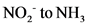There is a loss of oxygen atoms as well as gain of hydrogen atoms therefore, this reaction is a reduction reaction.

2.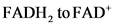There is a loss of hydrogen atoms therefore, this reaction is an oxidation reaction.

3.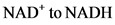There is a gain of hydrogen atoms therefore, this reaction is a reduction reaction.

4.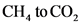There is a gain of oxygen atoms as well as a loss of hydrogen atoms therefore, this reaction is an oxidation reaction.

5.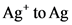There is a decrease in the oxidation number (that is, a gain of electrons) therefore, this reaction is a reduction reaction.

6.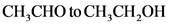There is a gain of hydrogen atoms therefore, this reaction is a reduction reaction.

7.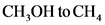There is a loss of oxygen atom therefore, this reaction is a reduction reaction.

8.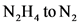There is a loss of hydrogen atoms therefore, this reaction is an oxidation reaction.

Explanation:
Oxidation and reduction processes have been identified for the given reactions by observing the changes from reactants to products during the reaction.
Oxidation-reduction concept:
The gain of oxygen or loss of hydrogen or loss of electrons in a molecule during a reaction is called oxidation. Conversely, the loss of oxygen or gain of hydrogen atoms or gain of electrons during a reaction is called a reduction.

Oxidation and reduction processes for the given species are tabulated below.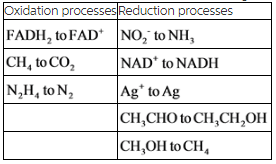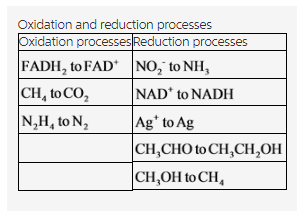Explanation:
The oxidation and reduction processes have been classified for the given reactions. There are three oxidation and five reduction reactions in the given reactions.